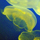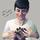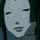## Social Question# How to find the highest point of a curve by 2nd order integration?

Asked by xichyu (222) April 14th, 2016

Areas under righting arm curves are carried out using 2nd order integration. Likewise, the
maximum righting arm is determined by a series of predictions and trials based on 2nd order
interpolation.

Observing members: 0Composing members: 0I’m not sure I understand the question but I’ll take a shot at it. :)

I’m not sure if I know of a way to use integration to find the highest point in a curve; the concept doesn’t make a whole lot of sense to me. But maybe you meant the second derivative?

The highest point of a curve is necessarily a place where the curve changes from “going up” to “going down.” That is, where the first derivative changes from positive to negative, meaning that at the precise highest point, the first derivative will be exactly zero.

Note, though, that the first derivative will also be zero at the low points of a curve, where the curve changes from “going down” to “going up.” This is where the second derivative comes in.

Once you’ve found a point where the first derivative is zero, take the second derivative of the equation and plug in the (x,y) value of the point you’ve found. If the result is negative, the point is a local maxima.

Note that some curves can have many local maxima, so a given local maximum is not necessarily the highest point in the curve. You’d have to find all the local maxima (all places where first derivative is equal to zero and the second derivative is negative) and then the highest point is the one with the largest y value.

Apologies if I’ve answered a question that is completely different from the one you’re meaning to ask.

Mariah (25863)“Great Answer” (0) Flag as…Is this “righting” as in a ship returning to the normal position? I’m guessing because you are asking a lot of boat questions. Building one or is your school using a lot of naval examples? You can probably find a good book or lecture on hydrostatics or “boat physics” to answer most of your questions
I’m also guessing you are already in one of these classes anyway.

ARE_you_kidding_me (19773)“Great Answer” (0) Flag as…xichyu (222)“Great Answer” (0) Flag as…